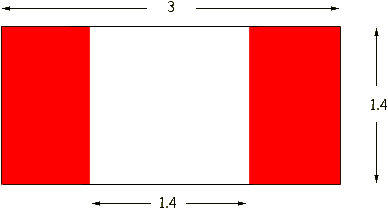Hi, I have a flag that's very similar to the canadian flag. Two smaller red areas and i larger white area. If I am given the length is 3 and the height is 1.4 how do I determine what the area of the two red areas is? Here's the answer but I can't figure out why they are multiplying 1.4 x 1.4? (3x1.4-1.4x1.4)divided by 2= 2.24 divided by 2 = 1.12 Hi Kim, From the answer you provide it looks like the white area must be a square.Thus 31.4 gives the area of the entire flag, 1.41.4 gives the area of the white portion which is to be ignored, and the division by 2 is to divide the red portion into its two parts. Penny